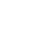Live chatGenerally factor models are used for describing and quantifying miscellaneous factors, affecting the return percentage for securities in the provided time frame. The range of use of the factor models is limited to making measurement and managerially supervising of different factors of economy, especially risks (energy price risk, interest risk etc.). A factor model could be also defined as a set of the security returns, separated into two categories. First one presents the security-correlated components and the second one reflects the components, not correlated across the securities.

Let us put the relevant economic factors together based on the common macroeconomic indicator. Supposedly all the factors are also firm-specific. They all come within a single company and are tightly related to it. Such way we get the simplest factor model – a single-factor model:

ri = αi + βi F + ei,

where ri is return on stock, bi – sensitivity of stock’s return to macroeconomic events, f is return to market index and ei is a random stock to return. The market return-generating factor is the only one in this model.Provided there are more than one common factors, as it occurs in real-life situations, we get a different factor model:

ri = αi + β1i F1 + β2i F2 + … + βki Fk + ei.

By means of the multifactor modeling we define the exposure of business to different macroeconomic risks. Security returns depend on a small number of common factors with different sensitivity of stocks. Portfolios are created for protection from possible risks.

To denote the risk premiums in the k-factors, λ1, λ2,…, λk are used. In other words, the expected return of factor portfolio 1 can be viewed as (rf + λ1), where rf is the risk-free rate. Let us assume the world is generated by a two-factor model and assume that we wish to replicate asset X, which has sensitivity β1x to the first factor and β2x to the second factor. Also we will assume that the primary securities being worked with are well-diversified portfolios themselves. That is why any idiosyncratic risk in this derivation will be ignored.

Limited time Offer

0
0
days
:
0
0
hours
:
0
0
minutes
:
0
0
seconds
Get 19% OFF

To replicate asset X`s factor sensitivities, we construct a portfolio with weight β1x on the first factor-replicating portfolio, weight β2x on the second factor-replicating portfolio and weight 1 - β1x - β2x on the risk-free asset. Hence, the expected return of the replicating portfolio is:

β1x (rf + λ1) + β2x (rf + λ2) + (1 - β1x - β2x ) rf = rf + β1x λ1 + β2x λ2.

By doing this for the k-th factor criteria, we get:

E(ri) = rf + β1i λ1 + β2i λ2 + ... + βki λk.

In case we require a two-factor model and the expected returns on assets will adjust such that there are no arbitrage profits (pair of portfolios must have identical expected return in equilibrium) to be made between broad portfolios, portfolios that have loadings on two factors must have expected return equal to the risk free plus the sum of the beta-weighted risk premiums for these factors. Formally, E(ri) = rf + β1i λ1 + β2i λ2, which is the statement of APT.

Stay ConnectedThe APT is based on returns of securities, described by the factor models, and non-existence of the arbitrage opportunities. Based on these ideas, one might derive the multifactor version of CAPM risk-expected return equation, expressing the expected returns of every single security in terms of factor beta function. The pros of APM are using of simple market portfolios, and the cons are the lack of the theoretical approach, found in CAPM.

0

Preparing Orders

0

Active Writers

0%

Positive Feedback

0

Support Agents

Limited offerget 15% off your 1st order with code first15